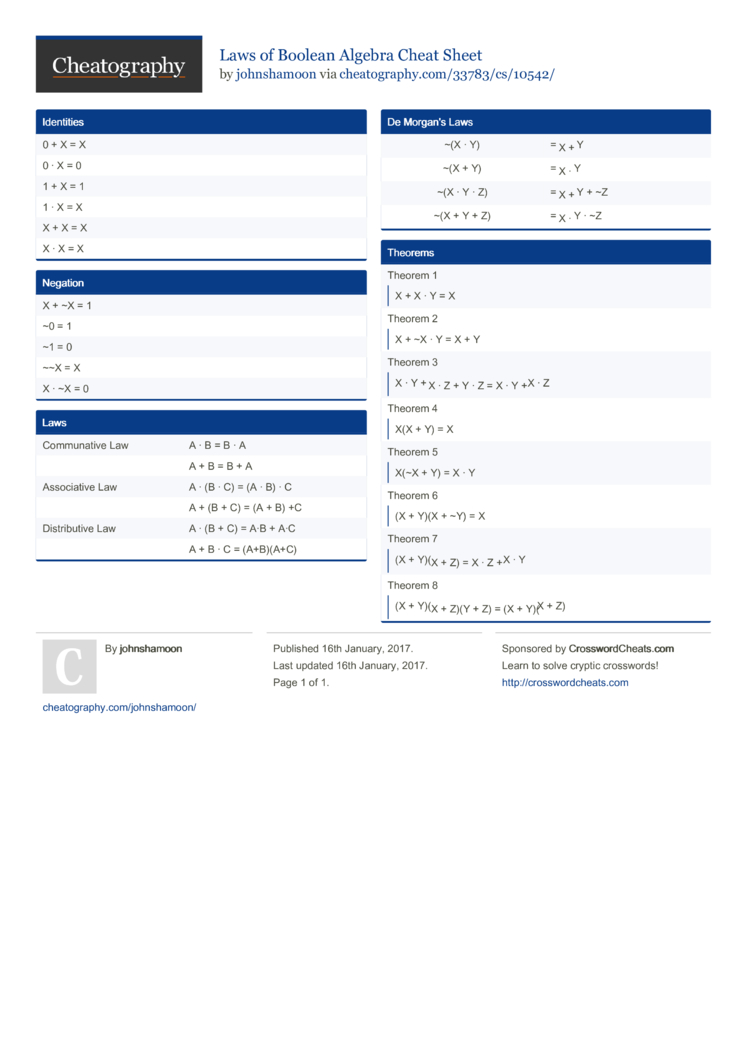# Laws of Boolean Algebra Cheat Sheet by johnshamoon

Axioms and Laws of Boolean Algebra

### Identities

 0 + X = X 0 · X = 0 1 + X = 1 1 · X = X X + X = X X · X = X

### Negation

 X + ~X = 1 ~0 = 1 ~1 = 0 ~~X = X X · ~X = 0

### Laws

 Commun­ative Law A · B = B · A A + B = B + A Associ­ative Law A · (B · C) = (A · B) · C A + (B + C) = (A + B) +C Distri­butive Law A · (B + C) = A·B + A·C A + B · C = (A+B)(A+C)

### De Morgan's Laws

 ~(X · Y) = ~X + ~Y ~(X + Y) = ~X · ~Y ~(X · Y · Z) = ~X + ~Y + ~Z ~(X + Y + Z) = ~X · ~Y · ~Z

### Theorems

 Theorem 1X + X · Y = X Theorem 2X + ~X · Y = X + Y Theorem 3X · Y + ~X · Z + Y · Z = X · Y + ~X · Z Theorem 4X(X + Y) = X Theorem 5X(~X + Y) = X · Y Theorem 6(X + Y)(X + ~Y) = X Theorem 7(X + Y)(~X + Z) = X · Z + ~X · Y Theorem 8(X + Y)(~X + Z)(Y + Z) = (X + Y)(~X + Z)1 Page
//media.cheatography.com/storage/thumb/johnshamoon_laws-of-boolean-algebra.750.jpg

PDF (recommended)